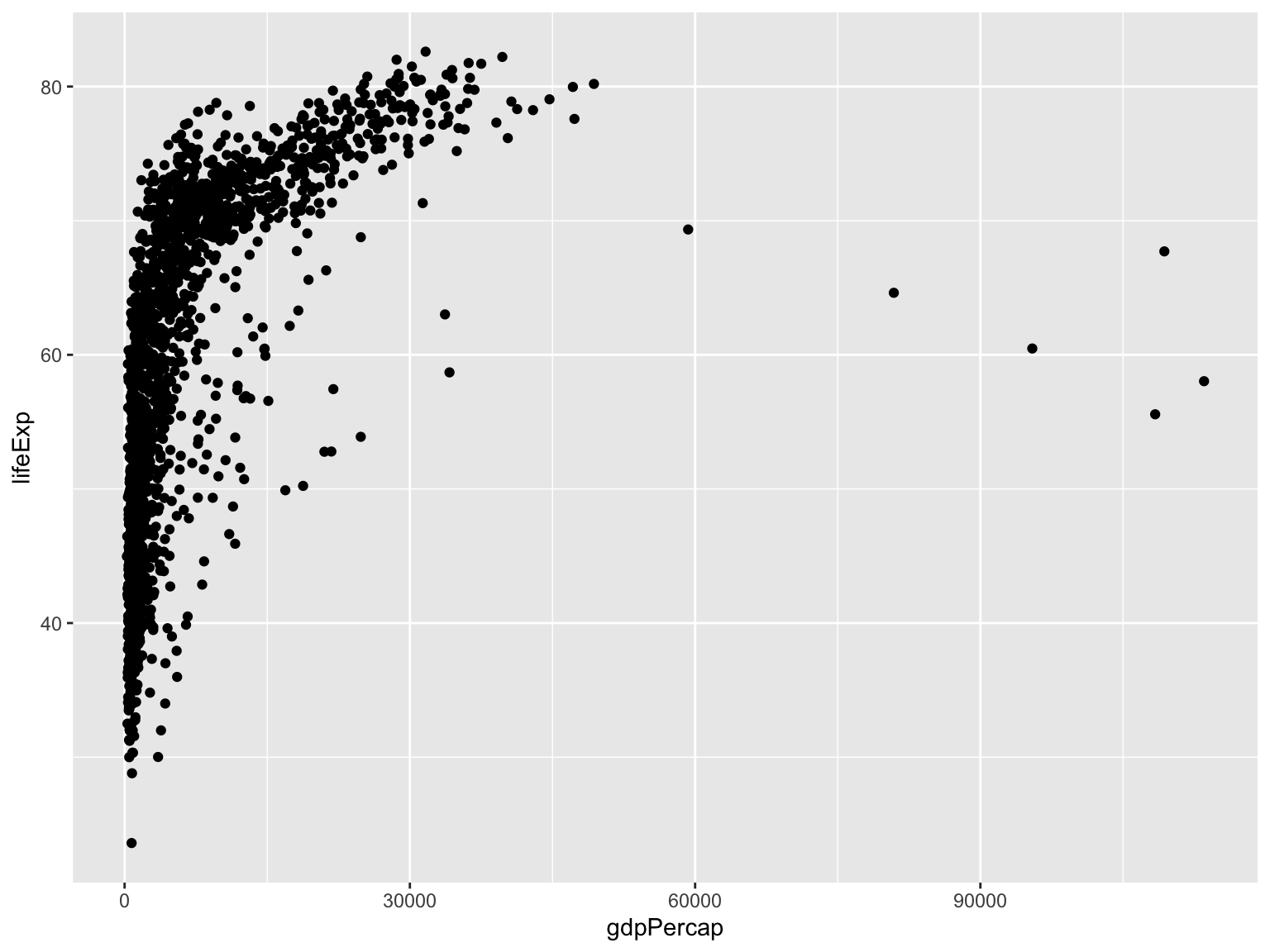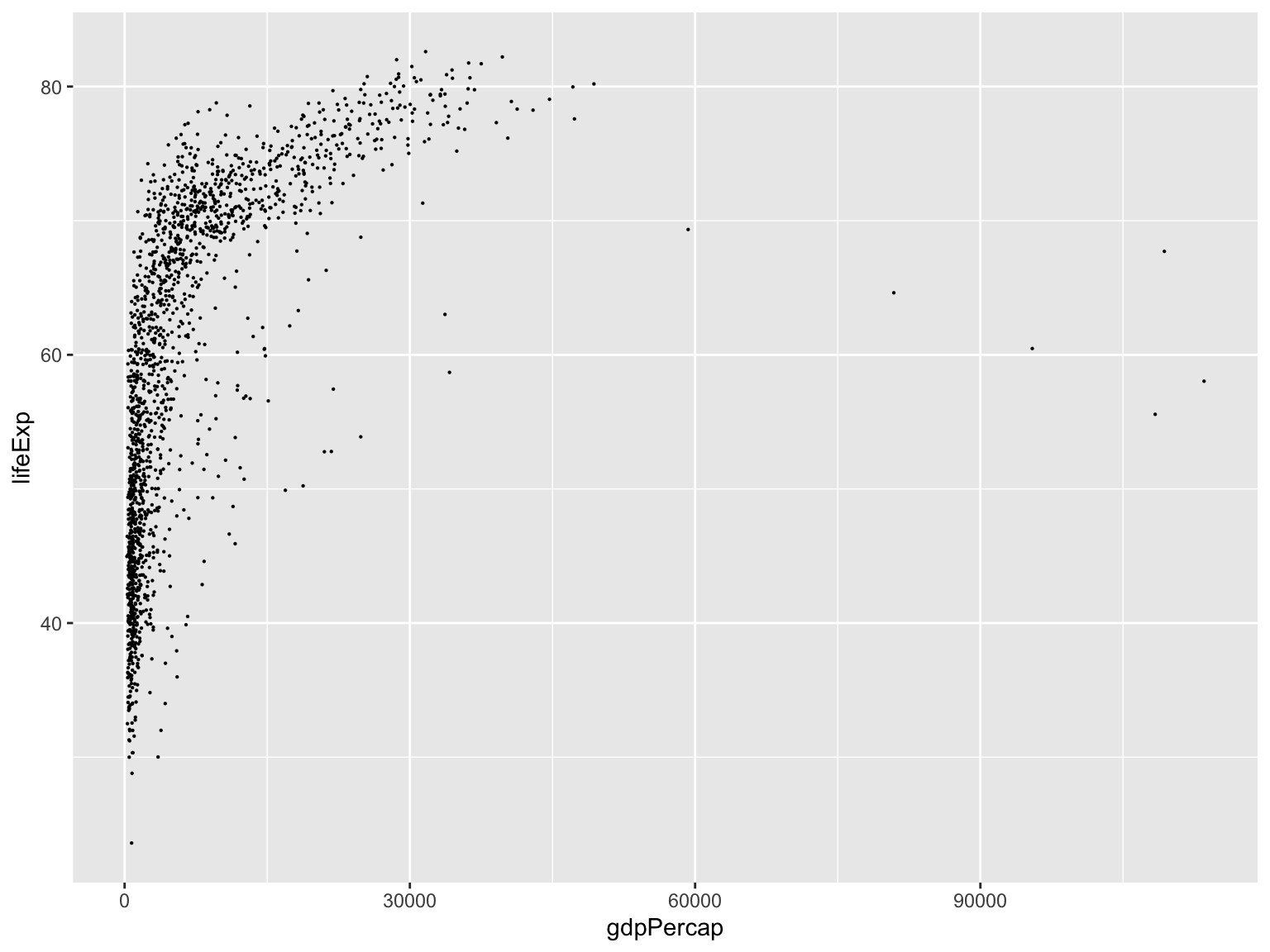# Plotting Tools

Wednesday July 10, 2019

# Last time: Data frames and `apply`

• Data frames are a representation of the “classic” data table in R: rows are observations/cases, columns are variables/features
• Each column can be a different data type (but must be the same length)
• `subset()`: function for extracting rows of a data frame meeting a condition
• `split()`: function for splitting up rows of a data frame, according to a factor variable
• `apply()`: function for applying a given routine to rows or columns of a matrix or data frame
• `lapply()`: similar, but used for applying a routine to elements of a vector or list
• `sapply()`: similar, but will try to simplify the return type, in comparison to `lapply()`
• `tapply()`: function for applying a given routine to groups of elements in a vector or list, according to a factor variable

# Part I

Plot basics: ggplot

# Plotting in R

There’s 2 major styles of plotting in `R`. You’ve already seen examples of base `R` plots include

• `plot()`: generic plotting function
• `hist()`: histogram
• and there are many more

But… the `R` community has come to embrace a new approach to visualization from the `ggplot2` package.

Extremely popular graphics library

# Base graphics

• Ugly, laborious, and verbose
• There are better ways to describe statistical visualizations.

# Why ggplot2?

• Follows a common grammar, just like any language.
• It defines basic components that make up a sentence. In this case, the grammar defines components in a plot.
• moreover, treats graphics like objects, mapping properties of your dataset to attributes of the graphic.
• Supports a continuum of expertise.
• encourages iterative creation of graphics
• Get started right away but with practice you can effortless build complex, publication quality figures.

# Basics: some terminology

• `ggplot`: The main function where you specify the dataset and variables to plot
• `geoms`: geometric objects
• `geom_point()`, `geom_bar()`, `geom_density()`, `geom_line()`, `geom_area()`
• `aes`: aesthetics
• `shape`, transparency (`alpha`), `color`, `fill`, `linetype`.
• `scales`: Define how your data will be plotted
• continuous, discrete, log

# Introduction to dataset

``````library(gapminder)
data(gapminder)``````

# ggplot intro: scatter plot

``````library(ggplot2)
ggplot(gapminder, aes(y = lifeExp, x = gdpPercap)) +
geom_point()``````# ggplot intro: basic structure

``````ggplot(data = gapminder, aes(y = lifeExp, x = gdpPercap)) +
geom_point()
p <- ggplot(data = gapminder, aes(y = lifeExp, x = gdpPercap))
p + geom_point()``````
• specific all “global” structure in the `ggplot` function (in this case `data` and variable mappings)
• add layers of geometric objects, statistical models, and panels.

# ggplot intro: change size

``````ggplot(gapminder, aes(y = lifeExp, x = gdpPercap)) +
geom_point(size = .1)````````````ggplot(gapminder, aes(y = lifeExp, x = gdpPercap, color = continent)) +